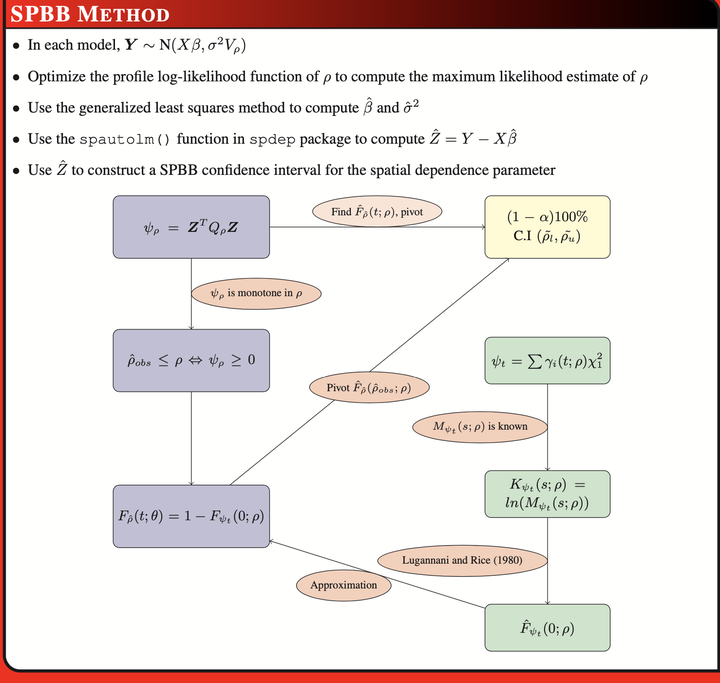We consider a class of statistical methods called the saddlepoint-based bootstrap (SPBB) method to make inferences about the spatial dependence parameter in the spatial regression model.

## Application

For example, in spatial econometrics, we can use the SPBB method to infer the significance of the spatial dependence parameter. We can use either the spatial autoregressive model, conditional autoregressive model, or spatial moving average models to fit the spatial lattice data.

## Method

We use the SPBB method to construct confidence intervals for a parameter whose estimator is a unique root of a quadratic estimating equation. In the SPBB method, we use the monotonicity of the quadratic estimating equation in parameter to relate the distribution of an estimator to the distribution of the respective quadratic estimating equation. Using the saddlepoint methods, it is then possible to accurately approximate the distribution of the estimator. Then confidence interval can be produced by pivoting the distribution of the estimator.